Courses

# Competition Level Test: Continuity And Differentiability- 3

## 13 Questions MCQ Test Mathematics For JEE | Competition Level Test: Continuity And Differentiability- 3

Description
This mock test of Competition Level Test: Continuity And Differentiability- 3 for JEE helps you for every JEE entrance exam. This contains 13 Multiple Choice Questions for JEE Competition Level Test: Continuity And Differentiability- 3 (mcq) to study with solutions a complete question bank. The solved questions answers in this Competition Level Test: Continuity And Differentiability- 3 quiz give you a good mix of easy questions and tough questions. JEE students definitely take this Competition Level Test: Continuity And Differentiability- 3 exercise for a better result in the exam. You can find other Competition Level Test: Continuity And Differentiability- 3 extra questions, long questions & short questions for JEE on EduRev as well by searching above.
QUESTION: 1

Solution:
QUESTION: 2

### The number of points at which the function f(x) = max. {a – x, a + x, b} – ∞ < x < ∞, 0 < a < b cannot be differentiable is

Solution:

A function is not differentiable at the points where it has sharp-corner point
Clearly above function has two sharp corner, so it is not differentiable at these two points,
Other than these points, function linear continuous, so it is diferentiable .
Hence above function is not differentiable at two points

QUESTION: 3

### The function f(x) is defined by f(x) =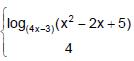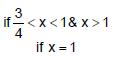Solution:

lt x1^+   f(x) = log(4x -3) (x2- 2x + 5)
= ln(x2 - 2x +5)/ln(4x-3)
lt(h → 0)   ln(1+h)2 - 2(1+h) + 5)/ln(4(1+h) - 3)
lt(h → 0)   ln(1+h2 + 2h - 2 -2h + 5)/ln(4 + 4h - 3)
ln(h → 0)    ln(4 + h2)/(1+4h)
Divide and multiply the denominator by 4h
ln(h → 0)    ln(4 + h2)/[((1+4h)/4h) * 4h]
As we know that (1+4h)/4h = 1
ln 4/(4*0)   = + ∞ (does not exist)
lt x→ 1^-   f(x) = log(4x -3) (x2- 2x + 5)
lt(h → 0)   ln(1-h)2 - 2(1-h) + 5)/ln(4(1-h) - 3)
lt(h → 0)   ln(1+h2 - 2h - 2 + 2h + 5)/ln(4 - 4h - 3)
ln(h → 0)    ln(4 + h2)/(1 - 4h)
Divide and multi[ly the denominator by (-4h)
ln(h → 0)    ln(4 + h2)/[((1+4h)/-4h) * (-4h)
As we know that (1-4h)/(-4h) = 1
ln 1/(-4*0)   = - ∞ (does not exist)

QUESTION: 4

Let f(x) =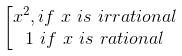then

Solution:
QUESTION: 5

A point where function f(x) is not continuous where f(x) = [sin [x]] in (0, 2π) ; is ([ * ] denotes greatest integer ≤ x)

Solution:
QUESTION: 6

Let f(x) = [n + p sin x], x ∈ (0, π), n ∈ I and p is a prime number. Then number of points where f(x) is not differentiable is (where [ * ] denotes greatest integer function)

Solution: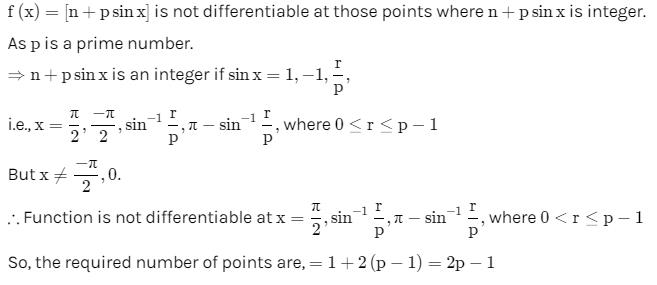QUESTION: 7

If f is a real-valued differentiable function satisfying |f(x) – f(y)| ≤ (x – y)2, x, y ∈ R and f(0) = 0, then f(1) equals

Solution:
QUESTION: 8

If f(x) =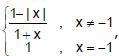, then f([2x]) is (where [ * ] represent greatest integer function)

Solution:
QUESTION: 9

The value of f(0), so that the function, f(x)=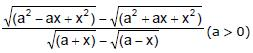becomes continuous for all x, is given by

Solution:

f(x)={(a2−ax+x2)−(a2+ax+x2)^1/2}/{(a+x -(a-x)1/2)1/2}
on rationalising, we get
lom(x → 0) a2 - ax + x2 -(a2 +ax + x2) * (a+x)1/2 + (a-x)1/2]/[(a+x)-(a-x) * (a2 - ax + x2)1/2 + (a2 + ax + x2)]
lim(x → 0) [-2ax * [(a)1/2 + (a)1/2]]/[2x * (a2)1/2 * (a2)1/2]
-a/(a)1/2 = -(a)1/2

QUESTION: 10

If f(x) =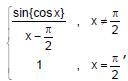then f(x) is (where { * } represents the fractional part function)

Solution:
QUESTION: 11

In order that function f (x) = (x + 1)cot x is continuous at x = 0, f (0) must be defined as

Solution:
QUESTION: 12

f is a continuous function on the real line. Given that x2 + (f(x) – 2) x –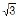. f(x) + 2– 3 = 0. then the value of f()

Solution:
QUESTION: 13

Given f(x)=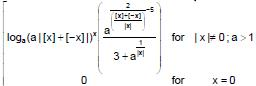then (where [*] represent the integral part function)

Solution: